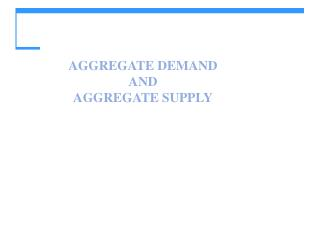DownloadDownload PresentationAGGREGATE DEMAND AND AGGREGATE SUPPLY

# AGGREGATE DEMAND AND AGGREGATE SUPPLY

Download Presentation## AGGREGATE DEMAND AND AGGREGATE SUPPLY

- - - - - - - - - - - - - - - - - - - - - - - - - - - E N D - - - - - - - - - - - - - - - - - - - - - - - - - - -
##### Presentation Transcript

1. AGGREGATE DEMAND ANDAGGREGATE SUPPLY

2. Production and Prices • Applied questions. • Why economies experience economic fluctuations? • How do policy actions by the government and the Federal Reserve affect output and prices? • To answer the applied questions we need to answer first the following theoretical questions: • How is GDP determined in the short-run? • How is the price level determined in the short-run?

3. Determination of GDP and Price level • In the short-run the aggregate price level are determined by the interaction of the aggregate supply and the aggregate demand. • Aggregate supply relates aggregate production to the price level • Aggregate demand relates aggregate expenditure to the price level

4. Aggregate Supply • Aggregate Supply Fundamentals • At any given time, the quantity of capital and the state of technology are fixed but the quantity of labor can vary - GDP = F(K,L,TFP) – • Thus, short-run fluctuations in employment are an important driving force of short-run fluctuations in GDP. • The wage rate that makes the quantity of labor demanded equal to the quantity supplied is the equilibrium wage rate and at that wage the level of employment is the natural rate of unemployment.

5. Aggregate Supply • Aggregate Supply Fundamentals • Aggregate supply with perfect price flexibility (also called by the book Long-run AS) • Aggregate supply where prices slowly adjust (the short-run aggregate supply curve)

6. Aggregate Supply • The LAS curve is vertical because potential GDP is independent of the price level. • Along the LAS curve all prices and wage rates vary by the same percentage so that relative prices and the real wage rate remain constant • (NEED TO EXPLAIN WHY THIS IS TRUE AND IT IS NOT EXPLAINED IN THE BOOK!)

7. Aggregate Supply • The SAS curve is upward sloping because: • A rise in the price level assuming wages fixes induces firms to hire more workers and to increase production • Can wages continue to be fixed?

8. Aggregate Supply • Along the SAS curve, real GDP might be above potential GDP… • … or below potential GDP • Because employment can be above or below the regular full employment level

9. Aggregate Supply • Changes in Aggregate Supply • When potential GDP increases, both the LAS and SAS curves shift rightward. • Potential GDP changes, for three reasons • Change in the quantity of capital (physical or human). • Advance in technology. • And, in general, anything that effects equilibrium employment levels.

10. Aggregate Demand • The quantity of real GDP demanded, Y, is the total amount of final goods and services produced in the United States that people, businesses, governments, and foreigners plan to buy. • This quantity is the sum of consumption expenditures, C, investment, I, government purchases, G, and net exports, X – M. That is: • Y = C + I + G + X – M.

11. Aggregate Demand • The Aggregate Demand Curve • Aggregate demand is the relationship between the quantity of real GDP demanded and the price level. • The aggregate demand (AD) curve plots the quantity of real GDP demanded against the price level.

12. Aggregate Demand • Buying plans depend on many factors and some of the main ones are: • The price level • Expectations • The world economy

13. Aggregate Demand • The AD curve slopes downward for two reasons • A wealth effect • Substitution effects

14. Aggregate Demand • Wealth effect A rise in the price level, other things remaining the same, decreases the quantity of real wealth (money, bonds, stocks, etc.). • To restore their real wealth, people increase saving and decrease spending, so the quantity of real GDP demanded decreases.

15. Aggregate Demand • International substitution effect A rise in the price level, other things remaining the same, increases the price of domestic goods relative to foreign goods, so imports increase and exports decrease, which decreases the quantity of real GDP demanded.

16. Macroeconomic Equilibrium • Short-Run Macroeconomic Equilibrium • Short-run macroeconomic equilibrium occurs when the quantity of real GDP demanded equals the quantity of real GDP supplied at the point of intersection of the AD curve and the SAS curve.

17. Macroeconomic Equilibrium • If total output and the price level are above equilibrium GDP, firms will have to decrease production and lower prices.

18. Macroeconomic Equilibrium • These changes bring a movement along the SAS curve toward equilibrium. • In short-run equilibrium, real GDP can be greater than or less than potential GDP.

19. Macroeconomic Equilibrium • Long-Run Macroeconomic Equilibrium • Long-run macroeconomic equilibrium occurs when real GDP equals potential GDP—when the economy is on its LAS curve.

20. Macroeconomic Equilibrium • Long-run equilibrium occurs where the AD and LAS curves intersect and results when the money wage has adjusted to put the SAS curve through the long-run equilibrium point.